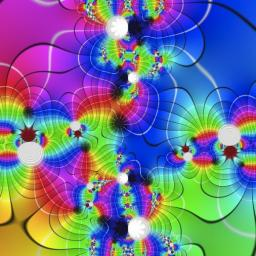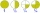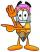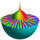# Product

Result of the product of the numbers 1, 2, 3, 1, 2, 0 is:

x =  0

### Step-by-step explanation:

1 . 2 . 3 . 1 . 2 . 0 = 0Did you find an error or inaccuracy? Feel free to write us. Thank you!## Related math problems and questions:

• Product increasedWhen the product of 2/3 and 6/10 is increased by 2/5, the result is?
• Product of the sum and differenceCalculate the product of the sum and difference of numbers -7 and -2.
• NumbersBy how many is the difference of numbers 8 and 34 less than its product?
• Two numbers and its productThe product of two numbers are 2/3. If on of them is 1/10, what is the other?
• How manzBy how many are the product of the numbers 328 and 7 greater than their sum?
• The product 2The product of two functions is 10. If one of them is 2 1/3, find the other one.
• Mistake in expressionWhile attempting to multiply the expression (2 - 5i)(5 + 2i) a student made a mistake. (2 - 5i)(5 + 2i) = 10 + 4i - 25i - 10i2 = 10 + 4(-1) - 25(-1) - 10(1) = 10 - 4 + 25 - 10 = 21 Complete the explanation and correct the error. Hint: The student inc
• MistakeNicol mistake when calculate in school. Instead of add number 20 subtract it. What is the difference between the result and the right result?
• The resultHow many times I decrease the number 1632 to get the result 24?
• DozenWhat is the product of 26 and 5? Write the answer in an Arabic numeral. Add up the digits. How many of this is in a dozen? Divide #114 by this
• FractionsSort fractions z1 = (6)/(11); z2 = (10)/(21); z3 = (19)/(22) by its size. Result write as three serial numbers 1,2,3.
• Evaluate 11Multiply the quotient of 6 and 2 by 3, then add 1. Add 1 and 6, then divide by 2 and multiply by 3. Divide 6 by the product of 2 and 3, then add 1.
• Written numberPlace+values x ten thousands =30 thousands
• DivisibilityIs the number 761082 exactly divisible by 9? (the result is the integer and/or remainder is zero)
• Write 3Write a real-world problem involving the multiplication of a fraction and a whole number with a product that is between 8 and 10, then solve the problem.
• The product 3The product of two numbers is 15 ⁵/₆. If one of the numbers is 5 ²/₃, find the other.
• JakubJakub multiplied 11 times the number 100 by ten and then divided the result ten times by 10. What number did he get?